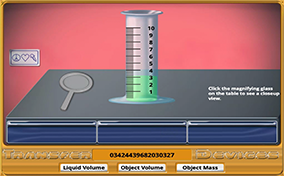# Volume-DensityIs this piece of jewelry pure gold or simply gold plating over some cheaper metal? That’s a question faced by many people when looking at a ring or bracelet. One of the best ways to make this determination is to find the density of the piece of jewelry. However, to find density, the volume of the substance must be known, and many objects have irregular shapes where easy measurements with rulers are not possible. Your challenge is to find the mass and volume of an irregular solid and then determine the object’s density.

### What is an irregular solid?

Any solid that does not have a shape easily measured by its length, width, height, and depth.

### What are SI units?

SI units are standard units of measure of seven specific quantities as defined by the International System of Units (SI). The seven quantities are time, length, mass, electric current, temperature, amount of a substance, and luminous intensity.

### What is mass?

Mass is the amount of matter in an object or substance.

### What are some common units for measuring mass?

The metric unit for measuring mass is the gram. The SI unit for measuring mass is the kilogram. Any metric prefix attached to the base gram unit is appropriate for measuring the mass of an object or substance. For instance, mg, cg, or ng.

### What is volume?

Volume is the amount of space occupied by an object or substance.

### What are some common units for measuring volume?

The metric unit for measuring volume is the liter. The SI unit for measuring volume is the cubic meter (m3). Any metric prefix attached to the base liter unit is appropriate for measuring the volume of an object or substance. For instance, mL, cL, or nL. Any metric prefix attached to the base SI unit is appropriate for measuring the volume of an object or substance. For instance, cm3, mm3, or km3,

### What is density?

Density is a measure of the compactness of an object or substance. To find the density of an object, the mass of the object is divided by the volume of the object.

### What are some common units for measuring density?

The units for density are any standard unit of mass over any standard unit of volume. For instance, grams/cubic centimeter or kilograms per liter.

### What is an intensive property?

A physical property that does not depend on the amount of a substance present. For instance, temperature, density, and the hardness of an object do not depend on how much of the object is present.

### What is an extensive property?

A physical property that does depend on the amount of a substance present. For instance, the mass and the length of a substance depend on how much of the substance is present. If more of a substance is present, then the substance’s mass changes.